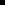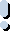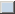##### Option 5.2.3. Retrieval Mode and Sample Interval for Historian Lines

If you select an Historian line type, you have different sampling options available for viewing raw data collected by Historian.

Select a sampling option for Historian lines:

1. (If the Line Display dialog box is not open) Open the Line Display dialog box.

1. Select an Historian line in the Lines grid.

2. Click .

The Line Display dialog box opens.

1. Select a Retrieval mode and Sample interval.Retrieval mode Default Sample Interval Enable Sample interval

 Retrieval mode Following are retrieval mode options. Raw Interpolated Minimum Raw Standard Deviation Maximum Standard Deviation Lab Count Raw Average Raw Total Average Total Trend

Note: The Sample Interval fields are enabled, unless you choose the Raw retrieval mode.

 Sample Mode Plotted Value Multiple data points along a vertical line. The arithmetic average of the good data quality raw points in the interval. This is useful only when a sufficient number of raw samples are collected. The minimum value in the interval. It may be a raw value or an interpolated value. The minimum must be a good data quality sample See "Understanding Qualities" in the Historian Electronic Books for more information about how Historian determines data quality. The maximum value in the sample interval. It may be a raw or an interpolated value. The maximum must be a good data quality sample. Lab Sampling was designed to match the way FIX32 and iFIX classic historian (HTA/HTC) returned data. Any value returned is an actual collected raw value; the data value is never interpolated. Interval timestamps are the same as in interpolated sampling; it is the values that can be different. Since lab sampling will return real collected values, it is more accurate when a sufficient number of raw samples are stored. Use interpolated sampling for highly compressed data. The average of the raw samples, but there is special logic for time weighting and for computing the value at the start of the interval. This is useful for computing an average on compressed data. Returns the raw minimum and raw maximum value for each specified interval. Use the Trend sampling mode to maximize performance when retrieving data points for plotting. A start time, end time, and an interval or number of samples must be specified. Trend sampling always returns an even number of samples, rounded up when necessary. If for instance, you request one sample in a specified time interval, Trending returns two samples. The graphical output from a request for seen samples is the same as the output for eight samples. Compression requires interpolation. Interpolation is done to produce an evenly spaced list of the most likely real-world values. Even if you are not using compression, you can use interpolation if you want samples spaced on intervals other than the collection rate. If you only want actual collected values to be returned, use Lab sampling. Typically, you use interpolated queries when data is not collected on a set time schedule, or if you want to see the results returned in an interval that is less than the collection rate. For example, these instances show when you can use interpolated mode to make evenly spaced out values:An Historian tag is collected as unsolicited. In this case, we really do not know what the time interval is between collected values.The Historian deadband and/or archive compression for a tag results in non-evenly spaced collection intervals.An Historian tag is collected once per 8-hour shift, but you want to see it displayed in 1 hour intervals with a linear slope between points. Retrieves the arithmetic standard deviation of raw values for each calculation interval. Retrieves the time-weighted standard deviation for each calculation interval. This is useful for computing a standard deviation on compressed data. The number of raw samples in the interval. This only addresses the count and not values or qualities of the samples. Count is useful for analyzing the distribution of the raw data samples. Retrieves the arithmetic sum of raw values for each interval. Use this mode to compute an accurate total when a sufficient number of raw samples are collected Retrieves the time-weighted total for each calculation interval. The collected value must be a rate. This calculation mode determines a count from the collected rate.

 Default Sample Interval Enable

A Default Sample Interval Enable checkbox has been added to the Trend control's Line Display dialog box.

When Default Sample Interval Enable is checked, retrieving 1000 samples for an Historian line is now maintained even when some portion of the trend chart is zoomed and the X-axis span is changed dynamically.

 Sample IntervalNote: Sample Interval is enabled when Default Sample Interval Enable is clear.

Define a time period for the Sample interval. The Sample interval is the time interval over which the selected Retrieval mode calculates or combines data to obtain a single data point for an Historian line.

1. Enter a value that represents the number of time units.

2. Select a time unit.

Example

Enter a value of 5 and select a time unit of second to retrieve a value from Historian every five seconds.

Result: Historian sends values for Trending to display based on the Retrieval mode and the amount of time you specify for the Sampling interval.

Notes:If Auto update is checked for this line, changing the chart's Auto update interval sets the time between data updates for that line. This interval does not change the shape of the line or the number of data points represented.The time interval between successive chart redraws is set by the Runtime refresh rate . This interval only affects the rate at which the entire chart is refreshed for new data.Important: You should configure a Sample interval that will limit the number of data points displayed on any given Historian line to approximately 1000.

Example:

If you have the X-axis configured to show two days of data (Duration is 02:00:00) at a Sample interval of one second (00:00:01), you will have 172,800 point to plot. However, the server limit is approximately 5,000 data points. So for a chart has a duration of two days, a reasonable Sample interval would be two minutes (00:02:00) or higher, i.e. 1,440 data points to plot across the chart.

 Methods to configure X-axis Timing.Review how Trending calculates interval start times. Option 7.1. Configure Basic Chart Properties
 SetLineCompression Method Step 4.2.7.4. Historian Line Configuration. Option 5.2. Trend Line Compression or Sample Type.